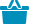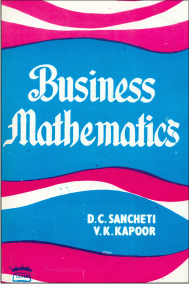Cart#### Quick Overview

Authored By

Publisher: Sultan Chand & Sons

Publishing Year: 2019

Size: 22.00 x 14.00

ISBN: 81-8054-538-2

MRP: 595.00

Availability: In Stock

₹595.00

The chief merit of the book is its simplicity. The book has 989 illustrations, 112 diagrams and 1149 exercises with answers for practice.

• Logical Statements and Truth Tables
• Theory of Sets
• Boolean Algebra
• Real Number Systems
• Groups, Ring and Field
• Indices and Surds
• Logarithms
• Equations : Linear Quadratic, Cubic and Higher Order
• Permutations and Combinations
• Binomial Theorem
• Mathematical Induction, Sequence and Series
• Arithmetic and Geometric Progressions
• Convergence and Divergence of Series
• Circular Functions and Trigonometry
• Coordinate Geometry
• Functions, Limits and Continuity
• Differential Calculus
• Integral Calculus
• Vector Algebra
• Matrix Algebra. Applications to Business and Economics
• Linear  Programming
• Probability
• Tables
• Index

ISBN13: 978-81-8054-538-2

Weight: 1150.00

Edition: 11th Revised Edition

Language: English

Title Code: 552

#### Author

Authored By : Kapoor V.K., Sancheti D.C.